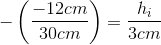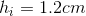# MCAT Physical : Thin Lens Equation

## Example Questions

### Example Question #4 : Optics

A virtual image is formed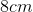from a convex mirror with a focal length of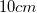. How far from the mirror is the object that created this image?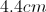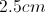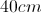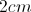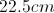Explanation:

Use the equation: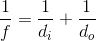Focal length is negative for convex mirrors, and image distance is negative for virtual images. We are given these values in the question, allowing us to calculate the object distance.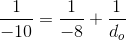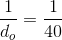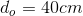### Example Question #1 : Thin Lens Equation

How far from a converging lens must an object be placed to produce an image that is NOT real and inverted? Given the answer as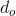in terms of the focal length,.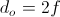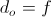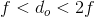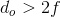Explanation:

When an object is placed a distance from a converging lens or mirror that is equal to the focal length, no image is produced. To test this out, stand in front of a single concave mirror and continue to back up until you no longer see an image. Once you've reached this point, you will be standing one focal length away from the mirror.

When the object is less than one focal length away from the converging lens/mirror, the image will be virtual and upright.

If you don't have these trends committed to memory, you can derive them from the equation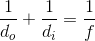.

When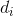is a negative integer the image is virtual, and when it is a positive integer the image is real.

### Example Question #6 : Optics

A certain farsighted person cannot focus on objects closer to his eyes than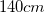. What focal length eyeglass lenses are needed in order to focus on a newspaper held at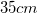from the person's eyes, if the glasses are worn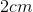from his eyes?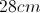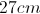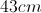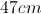Explanation:

First we need the object and image distances away from the eyeglass lenses. Here, the newspaper is the object and thefocal point is where the image needs to be located. Find the distance from the object to the lens, and the distance of the image to the lens, by subtracting out the distance from the lens to the eye.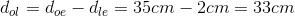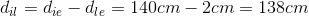Now apply the thin lens equation to determine focal length.Recall that if the image is on the same side of the lens as the object, then image distance is negative.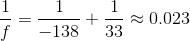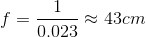### Example Question #1 : Optics

An object is placed 50cm in front of a concave mirror of radius 60cm. How far from the mirror is the image?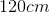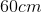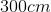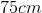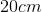Explanation:

Relevant equations: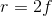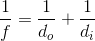Step 1: Find the focal length of the mirror.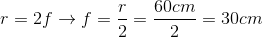Step 2: Plug this focal length and the object distance into the lens equation.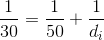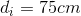### Example Question #8 : Optics

An object that is 3cm tall is placed 30cm from a convex spherical mirror of radius 40cm, along its central axis. What is the height of the image that is formed?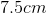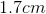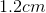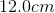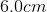Explanation:

Relevant equations: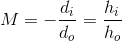Step 1: Find the focal length of the mirror (remembering that convex mirrors have negative focal lengths, by convention).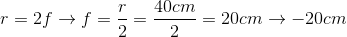Step 2: Find the image distance using the thin lens equation.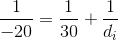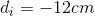Step 3: Use the magnification equation to relate the object distances and heights.